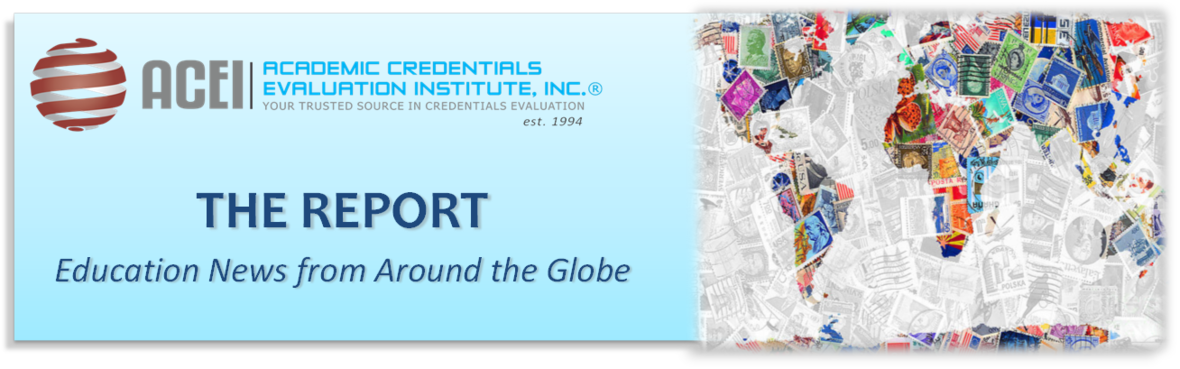# July 2019 - Beverly Hills, CA

ACADEMIC CREDENTIALS EVALUATION INSTITUTE, INC. (ACEI)
Is incorporated in the state of California (1994), and is dedicated to the advancement of international academic exchange and understanding through the dissemination of information on international academic credentials.

 table div table+table+table div table{width:100%;padding:0}table div table+table+table div table img{width:96.23%;padding:0;float:none}table div table+table+table div table td{width:100%;padding:0 1.88% 18px}/* styles */table div table+table+table+table+table div table{width:100%;padding:0}table div table+table+table+table+table div table table{padding:0;float:left!important;width:37.924%!important}table div table+table+table+table+table div table table+table td,table div table+table+table+table+table div table td{padding-left:0;padding-right:0}table div table+table+table+table+table div table table td{padding-left:0;padding-right:20px}table div table+table+table+table+table div table table+table{float:left!important;width:62.076%!important}/* styles */
 table div table+table+table+table+table+table+table div table{width:100%;padding:0}table div table+table+table+table+table+table+table div table img{width:96.23%;padding:0;float:none}table div table+table+table+table+table+table+table div table td{width:100%;padding:0 1.88% 18px}/* styles */# News Around the world

## Our round up of education news from around the world includes stories on female empowerment and inclusion of gender perspectives in school curriculum, assimilating children traumatized by war and conflict into communities through education, seeking solution to prevent migration of youth from eastern to western Europe by encouraging rapid reforms, and the continued growth of online and executive education offered through collaboration between private tech companies and accredited universities.

 table div table+table+table+table+table+table+table+table+table+table div table{width:100%;padding:0}table div table+table+table+table+table+table+table+table+table+table div table img{width:96.23%;padding:0;float:none}table div table+table+table+table+table+table+table+table+table+table div table td{width:100%;padding:0 1.88% 18px}/* styles */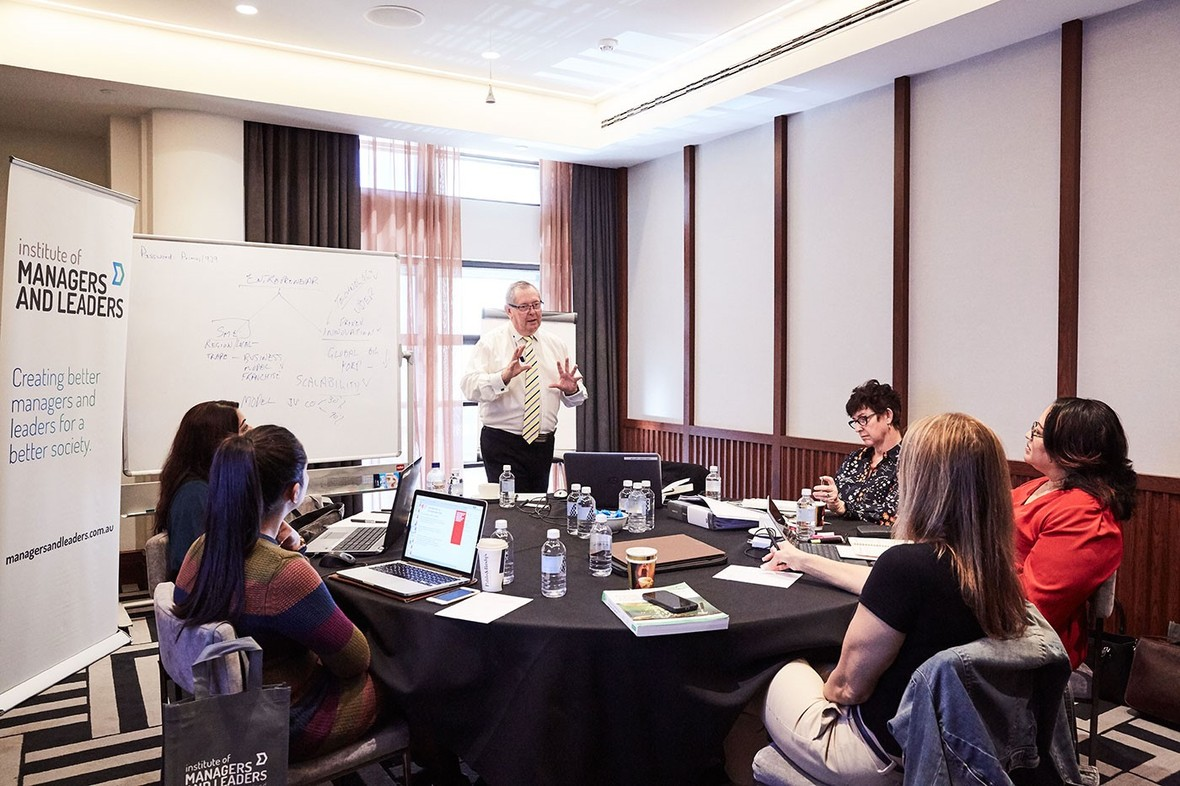# Universal Business School Sydney Unveils New MBA Program

 table div table+table+table+table+table+table+table+table+table+table+table+table+table div table{width:100%;padding:0}table div table+table+table+table+table+table+table+table+table+table+table+table+table div table img{width:96.23%;padding:0;float:none}table div table+table+table+table+table+table+table+table+table+table+table+table+table div table td{width:100%;padding:0 1.88% 18px}/* styles */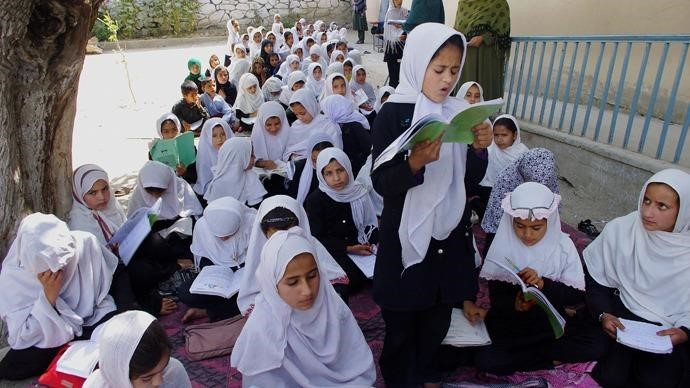table div table+table+table+table+table+table+table+table+table+table+table+table+table+table+table+table div table{width:100%;padding:0}table div table+table+table+table+table+table+table+table+table+table+table+table+table+table+table+table div table img{width:96.23%;padding:0;float:none}table div table+table+table+table+table+table+table+table+table+table+table+table+table+table+table+table div table td{width:100%;padding:0 1.88% 18px}/* styles */Photo credit: AP

# Looks to UK, Australia, and Canada

Caught in the crossfire of the US-China trade war, Chinese students are looking for alternative study destinations—which threatens to turn off an important source of revenue for American universities. To read more, click here.

 table div table+table+table+table+table+table+table+table+table+table+table+table+table+table+table+table+table+table+table div table{width:100%;padding:0}table div table+table+table+table+table+table+table+table+table+table+table+table+table+table+table+table+table+table+table div table img{width:96.23%;padding:0;float:none}table div table+table+table+table+table+table+table+table+table+table+table+table+table+table+table+table+table+table+table div table td{width:100%;padding:0 1.88% 18px}/* styles */# Germany warns the East: Speed Up Reforms or Risk Migration of its Youth

Germany’s minister of state for Europe delivered a blunt message to a gathering of the Eastern Partnership, organized in the Georgian Black Sea city of Batumi on July 11, 2019): unless reforms in Eastern Europe speed up, its young people will leave to find a better life in Western Europe. To read more, click here.

 table div table+table+table+table+table+table+table+table+table+table+table+table+table+table+table+table+table+table+table+table+table div table{width:100%;padding:0}table div table+table+table+table+table+table+table+table+table+table+table+table+table+table+table+table+table+table+table+table+table div table img{width:96.23%;padding:0;float:none}table div table+table+table+table+table+table+table+table+table+table+table+table+table+table+table+table+table+table+table+table+table div table td{width:100%;padding:0 1.88% 18px}/* styles */# Central European University: The Saga Continues

Students at Hungary’s Central European University wonder if they will be allowed to stay in the country after the government’s education reforms that has forced the University to relocate to Vienna, Austria. To read more, click here.

 table div table+table+table+table+table+table+table+table+table+table+table+table+table+table+table+table+table+table+table+table+table+table+table+table div table{width:100%;padding:0}table div table+table+table+table+table+table+table+table+table+table+table+table+table+table+table+table+table+table+table+table+table+table+table+table div table img{width:96.23%;padding:0;float:none}table div table+table+table+table+table+table+table+table+table+table+table+table+table+table+table+table+table+table+table+table+table+table+table+table div table td{width:100%;padding:0 1.88% 18px}/* styles */# On-line Executive Education

Mumbai is home to Eruditus, a start-up that its co-founder hopes will earn unicorn status (privately held companies with a valuation of \$1bn or more). Venture capitalist Sequoia India and Bertelsmann India Investments have invested in the company, which is shaking up executive education by delivering courses across the world in partnership with leading business schools. To read more, click here.

 table div table+table+table+table+table+table+table+table+table+table+table+table+table+table+table+table+table+table+table+table+table+table+table+table+table+table+table div table{width:100%;padding:0}table div table+table+table+table+table+table+table+table+table+table+table+table+table+table+table+table+table+table+table+table+table+table+table+table+table+table+table div table img{width:96.23%;padding:0;float:none}table div table+table+table+table+table+table+table+table+table+table+table+table+table+table+table+table+table+table+table+table+table+table+table+table+table+table+table div table td{width:100%;padding:0 1.88% 18px}/* styles */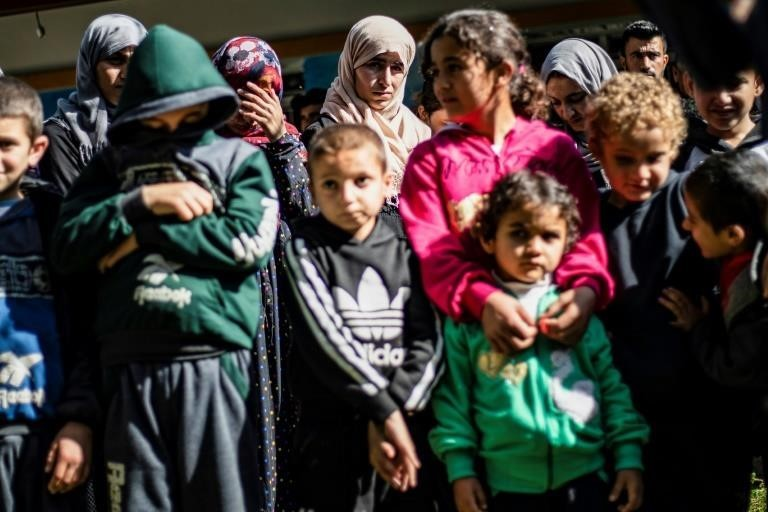# Minority children Traumatized by Ghosts of IS Captivity

Brainwashed and broken, the Islamic State group's youngest victims are struggling to recover from years of jihadist captivity as they return to their own traumatized minority communities in Iraq. To read more, click here.

 table div table+table+table+table+table+table+table+table+table+table+table+table+table+table+table+table+table+table+table+table+table+table+table+table+table+table+table+table+table+table div table{width:100%;padding:0}table div table+table+table+table+table+table+table+table+table+table+table+table+table+table+table+table+table+table+table+table+table+table+table+table+table+table+table+table+table+table div table img{width:96.23%;padding:0;float:none}table div table+table+table+table+table+table+table+table+table+table+table+table+table+table+table+table+table+table+table+table+table+table+table+table+table+table+table+table+table+table div table td{width:100%;padding:0 1.88% 18px}/* styles */# Integrating Gender Perspective into the Curriculum

Lebanon’s Ministry of Education and its Center for Educational Research and Development (CRDB) recently signed a partnership agreement with the Lebanese NGO ABAAD - Resource Centre for Gender Equality to integrate gender perspective into the country’s public-school curriculum. To read more, click here.

 table div table+table+table+table+table+table+table+table+table+table+table+table+table+table+table+table+table+table+table+table+table+table+table+table+table+table+table+table+table+table+table+table+table div table{width:100%;padding:0}table div table+table+table+table+table+table+table+table+table+table+table+table+table+table+table+table+table+table+table+table+table+table+table+table+table+table+table+table+table+table+table+table+table div table img{width:96.23%;padding:0;float:none}table div table+table+table+table+table+table+table+table+table+table+table+table+table+table+table+table+table+table+table+table+table+table+table+table+table+table+table+table+table+table+table+table+table div table td{width:100%;padding:0 1.88% 18px}/* styles */# Passes Law for National Research Misconduct Agency

After several high-profile cases, the country’s government is creating a board to oversee and investigate all serious allegations of scientific misconduct. To read more, click here

 table div table+table+table+table+table+table+table+table+table+table+table+table+table+table+table+table+table+table+table+table+table+table+table+table+table+table+table+table+table+table+table+table+table+table+table+table div table{width:100%;padding:0}table div table+table+table+table+table+table+table+table+table+table+table+table+table+table+table+table+table+table+table+table+table+table+table+table+table+table+table+table+table+table+table+table+table+table+table+table div table img{width:96.23%;padding:0;float:none}table div table+table+table+table+table+table+table+table+table+table+table+table+table+table+table+table+table+table+table+table+table+table+table+table+table+table+table+table+table+table+table+table+table+table+table+table div table td{width:100%;padding:0 1.88% 18px}/* styles */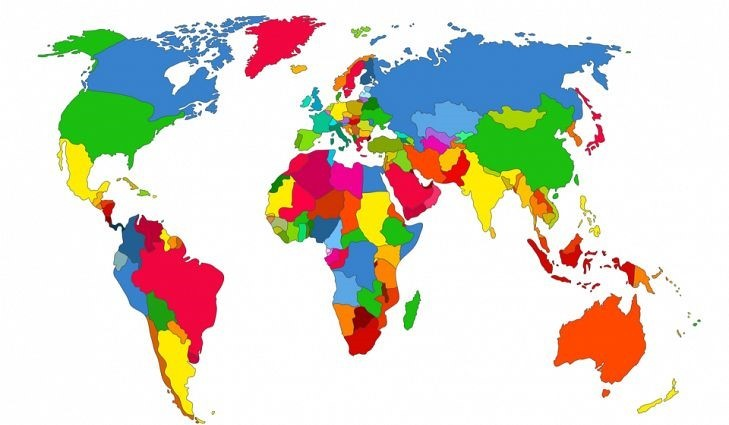# of Young Degree Holders to Reach 300 Million by 2030

==========
In an extensive analysis, the OECD has examined the fast-changing global higher education landscape over the past 10 years and changes that can be expected in the coming decade. It forecasts that the number of young people with a degree will reach 300 million in OECD and G20 countries by 2030 and highlights challenges of access, cost, quality and relevance. To read more, click here.

 table div table+table+table+table+table+table+table+table+table+table+table+table+table+table+table+table+table+table+table+table+table+table+table+table+table+table+table+table+table+table+table+table+table+table+table+table+table+table+table div table{width:100%;padding:0}table div table+table+table+table+table+table+table+table+table+table+table+table+table+table+table+table+table+table+table+table+table+table+table+table+table+table+table+table+table+table+table+table+table+table+table+table+table+table+table div table img{width:96.23%;padding:0;float:none}table div table+table+table+table+table+table+table+table+table+table+table+table+table+table+table+table+table+table+table+table+table+table+table+table+table+table+table+table+table+table+table+table+table+table+table+table+table+table+table div table td{width:100%;padding:0 1.88% 18px}/* styles */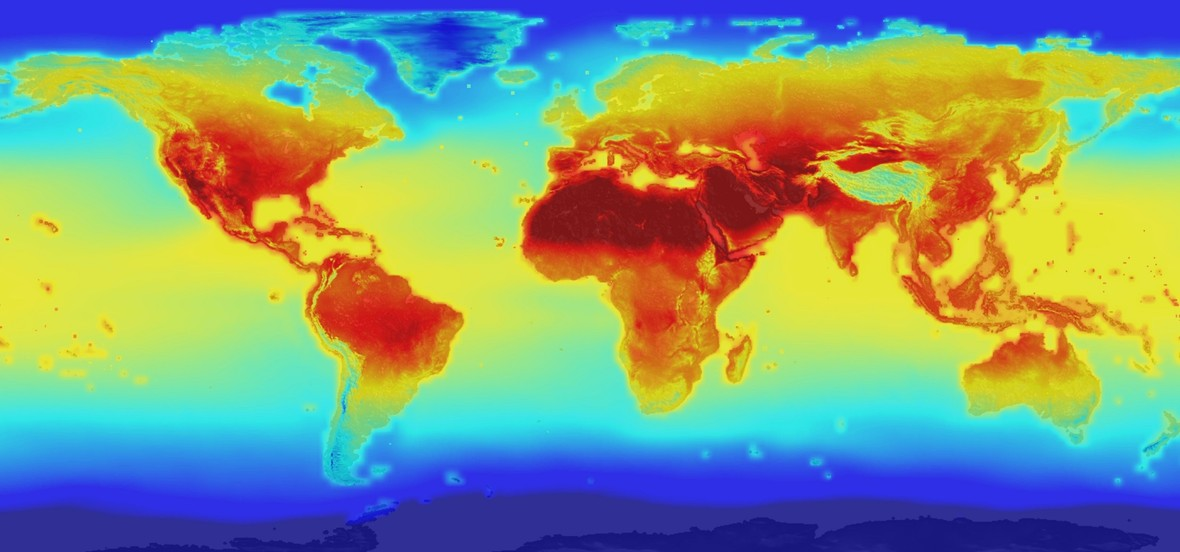# More than 7000 Universities Declare Climate Emergency

Networks representing more than 7,000 higher and further education institutions from six continents have announced that they are declaring a ‘climate emergency’ and agreed to undertake a three-point plan to address the crisis through their work with students. To read more, click here

 table div table+table+table+table+table+table+table+table+table+table+table+table+table+table+table+table+table+table+table+table+table+table+table+table+table+table+table+table+table+table+table+table+table+table+table+table+table+table+table+table+table+table div table{width:100%;padding:0}table div table+table+table+table+table+table+table+table+table+table+table+table+table+table+table+table+table+table+table+table+table+table+table+table+table+table+table+table+table+table+table+table+table+table+table+table+table+table+table+table+table+table div table img{width:96.23%;padding:0;float:none}table div table+table+table+table+table+table+table+table+table+table+table+table+table+table+table+table+table+table+table+table+table+table+table+table+table+table+table+table+table+table+table+table+table+table+table+table+table+table+table+table+table+table div table td{width:100%;padding:0 1.88% 18px}/* styles */## U.S.A.

The United States Department of Education is stepping up its scrutiny of whether colleges comply with federal reporting requirements regarding disclosures of foreign gifts and contracts. To find out, click here.

 table div table+table+table+table+table+table+table+table+table+table+table+table+table+table+table+table+table+table+table+table+table+table+table+table+table+table+table+table+table+table+table+table+table+table+table+table+table+table+table+table+table+table+table+table div table{width:100%;padding:0}table div table+table+table+table+table+table+table+table+table+table+table+table+table+table+table+table+table+table+table+table+table+table+table+table+table+table+table+table+table+table+table+table+table+table+table+table+table+table+table+table+table+table+table+table div table img{width:96.23%;padding:0;float:none}table div table+table+table+table+table+table+table+table+table+table+table+table+table+table+table+table+table+table+table+table+table+table+table+table+table+table+table+table+table+table+table+table+table+table+table+table+table+table+table+table+table+table+table+table div table td{width:100%;padding:0 1.88% 18px}/* styles */# Upcoming Events## Upcoming Events 2019:

IHE NOW
The Admissions Challenges Facing Private Colleges
August 16, 2019, San Francisco, CA
New Zealand International Education Conference & Expo
Navigating Our Changing World
August 7-8, 2019 in Auckland, New Zealand
European Association of International Educators (EAIE),
Annual Conference
September 24-27, 2019, Helsinki, Finland
NACAC 75th National Conference
September 26-28, 2019 in Louisville, Kentucky
Hispanic Association of Colleges and Universities 33rd Annual Conference
October 5-7, 2019 in Chicago, IL
AIEC (Australian International Education Conference) 2019
October 15-18, 2019 in Perth, Australia
Global Citizenship for Campus, Community, and Careers Network Conference
Crossing Borders and Boundaries
October 17–19, 2019 in San Antonio, Texas
Alliance for International Exchange Annual Conference
Shaping the Future
October 24-25, 2019 in Washington, DC
IVEC 2019
International Virtual Exchange Conference
October 25-26, 2019 in Tacoma, Washington
2019 CIEE Annual Conference
Opening Doors to Increase Diversity in Study Abroad
November 6-8, 2019 in Brooklyn, New York

 table div table+table+table+table+table+table+table+table+table+table+table+table+table+table+table+table+table+table+table+table+table+table+table+table+table+table+table+table+table+table+table+table+table+table+table+table+table+table+table+table+table+table+table+table+table+table+table+table div table{width:100%;padding:0}table div table+table+table+table+table+table+table+table+table+table+table+table+table+table+table+table+table+table+table+table+table+table+table+table+table+table+table+table+table+table+table+table+table+table+table+table+table+table+table+table+table+table+table+table+table+table+table+table div table img{width:96.23%;padding:0;float:none}table div table+table+table+table+table+table+table+table+table+table+table+table+table+table+table+table+table+table+table+table+table+table+table+table+table+table+table+table+table+table+table+table+table+table+table+table+table+table+table+table+table+table+table+table+table+table+table+table div table td{width:100%;padding:0 1.88% 18px}/* styles */table.module-49{width:84.72%;padding:0}table div table+table+table+table+table+table+table+table+table+table+table+table+table+table+table+table+table+table+table+table+table+table+table+table+table+table+table+table+table+table+table+table+table+table+table+table+table+table+table+table+table+table+table+table+table+table+table+table+table+table div table{width:84.72%;float:none;margin-left:auto;margin-right:auto;padding:0}table div table+table+table+table+table+table+table+table+table+table+table+table+table+table+table+table+table+table+table+table+table+table+table+table+table+table+table+table+table+table+table+table+table+table+table+table+table+table+table+table+table+table+table+table+table+table+table+table+table+table div table a{border:0 none;text-decoration:none}table div table+table+table+table+table+table+table+table+table+table+table+table+table+table+table+table+table+table+table+table+table+table+table+table+table+table+table+table+table+table+table+table+table+table+table+table+table+table+table+table+table+table+table+table+table+table+table+table+table+table div table img{width:100%!important;border:0 none;text-decoration:none}table div table+table+table+table+table+table+table+table+table+table+table+table+table+table+table+table+table+table+table+table+table+table+table+table+table+table+table+table+table+table+table+table+table+table+table+table+table+table+table+table+table+table+table+table+table+table+table+table+table+table div table td{width:100%;padding:0}/* styles */

# Digital Delivery of Evaluation Reports: SecurePathway©:

ACEI is a proud signatory of the Groningen Declaration Network which “seeks common ground in best serving the academic and professional mobility needs of citizens worldwide by bringing together key stakeholders in the Digital Student Data Ecosystem.” For the past two years, ACEI has committed itself to this shared mission through the introduction of SecurePathway©, an ACEI proprietary digital platform for the secure delivery of official evaluation reports and supporting educational credentials. For information on ACEI’s SecurePathway©.

Signing up with ACEI’s SecurePathway©, is free and does not cost the institutions any fees. To receive ACEI expert reports and certified academic credentials via SecurePathway©, complete this form.

*SecurePathway Registration Form*/* styles */ ACEI’s Advisory Services provide institutions and organizations looking at expanding their international student recruitment with customized solutions to help overcome enrollment challenges, uncover opportunities, and devise an innovative and holistic approach to international enrollment. For more information on ACEI’s Advisory Services and how we can assist you, please contact Yolinisse Moreno, Director of Programs & Communications at ymoreno@acei-global.org.
 table div table+table+table+table+table+table+table+table+table+table+table+table+table+table+table+table+table+table+table+table+table+table+table+table+table+table+table+table+table+table+table+table+table+table+table+table+table+table+table+table+table+table+table+table+table+table+table+table+table+table+table+table+table+table div table{width:100%;padding:0}table div table+table+table+table+table+table+table+table+table+table+table+table+table+table+table+table+table+table+table+table+table+table+table+table+table+table+table+table+table+table+table+table+table+table+table+table+table+table+table+table+table+table+table+table+table+table+table+table+table+table+table+table+table+table div table img{width:96.23%;padding:0;float:none}table div table+table+table+table+table+table+table+table+table+table+table+table+table+table+table+table+table+table+table+table+table+table+table+table+table+table+table+table+table+table+table+table+table+table+table+table+table+table+table+table+table+table+table+table+table+table+table+table+table+table+table+table+table+table div table td{width:100%;padding:0 1.88% 18px}/* styles *//* styles */ ACEI offers a comprehensive range of professional services that include: international credential evaluations; translations; consulting; and training. For more information on ACEI, please visit www.acei-global.org or contact: Tel: 1-310-275-3530; Email: acei@acei-global.org. ACEI was founded and incorporated in 1994 in the State of California and is a Charter and Endorsed Member of the Association of International Credential Evaluators (AICE).
 Like   Tweet   Pin   +1   in